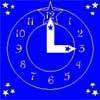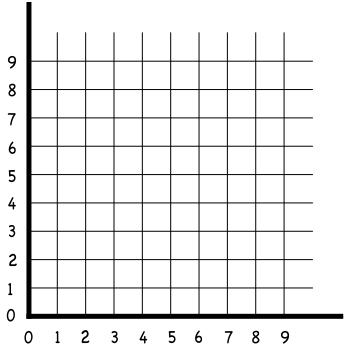#### You may also like### Great Squares

Investigate how this pattern of squares continues. You could measure lengths, areas and angles.### Hand Span

Use your hand span to measure the distance around a tree trunk. If you ask a friend to try the same thing, how do the answers compare?### Watch the Clock

During the third hour after midnight the hands on a clock point in the same direction (so one hand is over the top of the other). At what time, to the nearest second, does this happen?

# Drawing

##### Age 5 to 11Challenge Level

You will need squared paper for this activity.
Start by drawing a set of axes like this:Then, draw the following lines:
From (1,1) to (4,5)
From (4,5) to (8,8)
From (1,1) to (5,4)
From (5,4) to (8,8)

Then measure each of the four lines accurately.
What shape have you drawn?
Now, measure the distance from the bottom left to the top right of your picture (the long diagonal of the shape).
What is the length of the short diagonal?